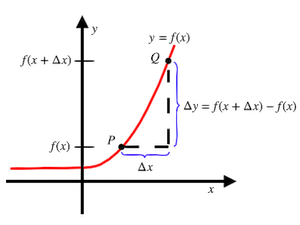# Precalculus - Introduction to Derivatives

## Introduction to Derivatives

• The instantaneous change of a function with respect to an independent variable is called the derivative of the function.
• The derivative of a function $y=f\left(x\right)$ with respect to $x$ is represented as $\frac{dy}{dx}$ or ${f}^{\text{'}}\left(x\right)$ or ${y}^{\text{'}}$ or ${y}^{\left(1\right)}$.
• Geometrically, the derivative of a function at a point is the slope of the tangent line on the curve of the function at that point.
• Mathematically, the derivative of a function $y=f\left(x\right)$ at a point  is defined as

$\underset{∆x\to 0}{\mathrm{lim}}\frac{f\left(x+∆x\right)-f\left(x\right)}{∆x}$, if the limit exists at the point  as shown in the figure.## Differentiation Rules

• Derivative of a Constant: If $c$ is any constant, then $\frac{d}{dx}\left(c\right)=0$.

Example: $\frac{d}{dx}\left(12\right)=0$

• Derivative of ${\left(\text{variable}\right)}^{\mathbf{\text{constant}}}$: $\frac{d}{dx}\left({x}^{n}\right)=n{x}^{n-1}$, where $n$ is a real number.

Example: $\frac{d}{dx}\left({x}^{4}\right)=4{x}^{4-1}=4{x}^{3}$.

• Derivative of Sum or Difference of functions: $\frac{d}{dx}\left(f\left(x\right)±g\left(x\right)\right)=\frac{d}{dx}f\left(x\right)±\frac{d}{dx}g\left(x\right)$

Example: $\frac{d}{dx}\left(12{x}^{2}+10{x}^{4}\right)$

$=\frac{d}{dx}\left(12{x}^{2}\right)+\frac{d}{dx}\left(10{x}^{4}\right)$

$=12\left(2x\right)+10\left(4{x}^{3}\right)$

$=24x+40{x}^{3}$

• Derivative of Quotient of two functions: $\frac{d}{dx}\left[\frac{f\left(x\right)}{g\left(x\right)}\right]=\frac{g\left(x\right)\frac{d}{dx}\left(f\left(x\right)\right)-f\left(x\right)\frac{d}{dx}\left(g\left(x\right)\right)}{{\left(g\left(x\right)\right)}^{2}}$

Example: $\frac{d}{dx}\left(\frac{x+1}{{x}^{2}}\right)$

$=\frac{\left({x}^{2}\right)\frac{d}{dx}\left(x+1\right)-\left(x+1\right)\frac{d}{dx}\left({x}^{2}\right)}{{\left({x}^{2}\right)}^{2}}$

$=\frac{-x-2}{{x}^{3}}$

• Derivative of Product of two functions: $\frac{d}{dx}f\left(x\right)·g\left(x\right)=f\left(x\right)\frac{d}{dx}g\left(x\right)+g\left(x\right)\frac{d}{dx}f\left(x\right)$

Example: $\frac{d}{dx}\left[\left(3{x}^{2}+1\right)\left({x}^{2}-1\right)\right]$

$=\left(3{x}^{2}+1\right)\frac{d}{dx}\left({x}^{2}-1\right)+$$\left({x}^{2}-1\right)\frac{d}{dx}\left(3{x}^{2}+1\right)$

$=\left(3{x}^{2}+1\right)\left(2x\right)+\left({x}^{2}-1\right)\left(6x\right)$

$=12{x}^{3}-4x$

• Derivative of the function of a function (Chain Rule): $\frac{d}{dx}f\left(g\left(x\right)\right)=\frac{d}{dx}f\left(g\left(x\right)\right)·\frac{d}{dx}g\left(x\right)$

Example: $\frac{d}{dx}{\left(3x-4\right)}^{3}$

$=3{\left(3x-4\right)}^{3-1}\frac{d}{dx}\left(3x-4\right)$

$=3{\left(3x-4\right)}^{2}\left(3\right)$

$=9{\left(3x-4\right)}^{2}$

• Derivative of Exponential Functions: $\frac{d}{dx}\left({e}^{x}\right)={e}^{x}$ and , where $a>0$ and $a\ne 1$.

Example: $\frac{d}{dx}\left({e}^{x}+{2}^{x}\right)$

• Derivative of Logarithmic Functions:  and , where $a>0$ and $a\ne 1$.

Example:

• Derivatives of Trigonometric Functions:
• Higher Order Derivatives: Differentiating a function more than once (successive derivatives of a function) results in higher order derivatives. The higher-order derivatives of a function $y=f\left(x\right)$ is mentioned below.
• First Derivative: $\frac{dy}{dx}={f}^{\text{'}}\left(x\right)$
• Second Derivative: $\frac{{d}^{2}y}{d{x}^{2}}=\frac{d}{dx}\left(\frac{dy}{dx}\right)={f}^{\text{'}\text{'}}\left(x\right)$
• ${n}^{th}$ derivative: $\frac{{d}^{n}y}{d{x}^{n}}=\frac{d}{dx}\left(\frac{{d}^{n-1}y}{d{x}^{n-1}}\right)={f}^{n}\left(x\right)$

Example.: The first and second derivatives of a function $y={x}^{5}+4{x}^{4}-3{x}^{3}$ are:

First derivative:

${y}^{\text{'}}=5{x}^{5-1}+4·4{x}^{4-1}-3·3{x}^{3-1}$

$=5{x}^{4}+16{x}^{3}-9{x}^{2}$

Second derivative:

${y}^{\text{'}\text{'}}=5·4{x}^{4-1}+16·3{x}^{3-1}-9·2{x}^{2-1}$

$=20{x}^{3}+48{x}^{2}-18x$

## Solved Examples

Example 1. Find the derivative of $f\left(x\right)={x}^{2}+5x$.

Solution: $f\left(x\right)={x}^{2}+5x$

${f}^{\text{'}}\left(x\right)=2x+5$

Example 2: Find $\frac{dy}{dx}$ if .

Solution:

Example 3: Find ${y}^{\text{'}}$ if $y=\left(2x+5\right)\left(5{x}^{2}-1\right)$.

Solution: $y=\left(2x+5\right)\left(5{x}^{2}-1\right)$

${y}^{\text{'}}=\left(2x+5\right)\left(10x\right)+\left(2\right)\left(5{x}^{2}-1\right)$

$=\left(20{x}^{2}+50x\right)+\left(10{x}^{2}-2\right)$

$=20{x}^{2}+50x+10{x}^{2}-2$

$=30{x}^{2}+50x-2$

Example 4: If $y=\frac{9}{\left(x-3\right)}$, find ${y}^{\text{'}}$ at .

Solution: $y=\frac{9}{\left(x-3\right)}$

${y}^{\text{'}}=\frac{0\left(x-3\right)-1\left(9\right)}{{\left(x-3\right)}^{2}}$

$=\frac{-9}{{\left(x-3\right)}^{2}}$

At ${y}^{\text{'}}=\frac{-9}{{\left(1-3\right)}^{2}}$$=\frac{-9}{{\left(-2\right)}^{2}}$$=-\frac{9}{4}$

Example 5: Find the slope of the tangent line to the curve $y={x}^{2}-4$ at the point .

Solution: The slope of the tangent line to a curve is its derivative. So, the slope of the tangent line to the curve $y={x}^{2}-4$ is

${y}^{\text{'}}=2x$

At ${y}^{\text{'}}=2×3$$=6$

Example 6: Find ${y}^{\text{'}}$ if $y={17}^{\sqrt{x}}$.

Solution: $y={17}^{\sqrt{x}}$

Let us apply the chain rule to directly find the derivative of the given function.

Example 7: Find ${f}^{\text{'}}\left(x\right)$ if .

Solution:

Let us apply the chain rule to directly find the derivative of the given function.

## Cheat Sheet

• The derivative ${f}^{\text{'}}\left(x\right)$ of a function $f\left(x\right)$ can be mathematically represented as ${f}^{\text{'}}\left(x\right)=\underset{∆x\to 0}{\mathrm{lim}}\frac{f\left(x+∆x\right)-f\left(x\right)}{∆x}$.
• In simple terms, the derivative of a function at a point is simply the slope of the tangent line on the curve of the function at that point.
• Some of the standard results of differentiation are mentioned below.
• $\frac{d}{dx}\left(\text{constant}\right)=0$
• $\frac{d}{dx}\left({x}^{n}\right)=n{x}^{n-1}$
• $\frac{d}{dx}\left({e}^{x}\right)={e}^{x}$
• , where $a>0$ and $a\ne 1$
• , where $a>0$ and $a\ne 1$
• $\frac{d}{dx}\left[f\left(x\right)±g\left(x\right)\right]=\frac{d}{dx}\left[f\left(x\right)\right]±\frac{d}{dx}\left[g\left(x\right)\right]$
• $\frac{d}{dx}\left[f\left(x\right)·g\left(x\right)\right]$$=f\left(x\right)·\frac{d}{dx}\left[g\left(x\right)\right]+g\left(x\right)·\frac{d}{dx}\left[f\left(x\right)\right]$
• $\frac{d}{dx}\left[\frac{f\left(x\right)}{g\left(x\right)}\right]=\frac{g\left(x\right)·\frac{d}{dx}\left[f\left(x\right)\right]-f\left(x\right)·\frac{d}{dx}\left[g\left(x\right)\right]}{{\left[g\left(x\right)\right]}^{2}}$
• $\frac{d}{dx}\left[f\left(g\left(x\right)\right)\right]=\frac{d}{dx}\left[f\left(g\left(x\right)\right)\right]·\frac{d}{dx}\left[g\left(x\right)\right]$ (Chain Rule)

## Blunder Areas

• Always be mindful that not all continuous functions are differentiable.
• It is helpful to examine the type of function based on its equation or graph to determine whether it is differentiable or not.
• Differentiation formulas, comprehensively discussed in other lessons, are helpful and quick ways to find the derivative of a function.